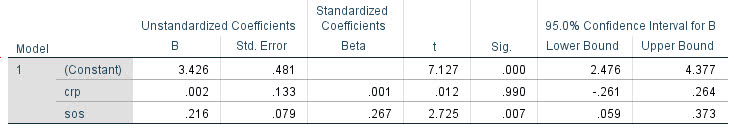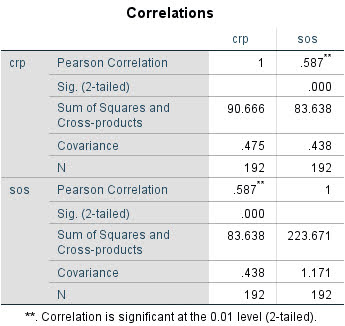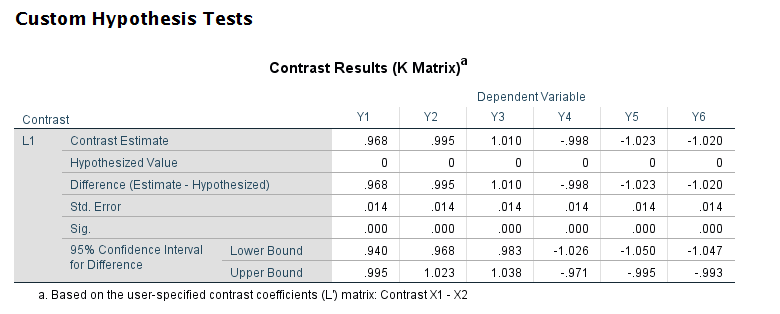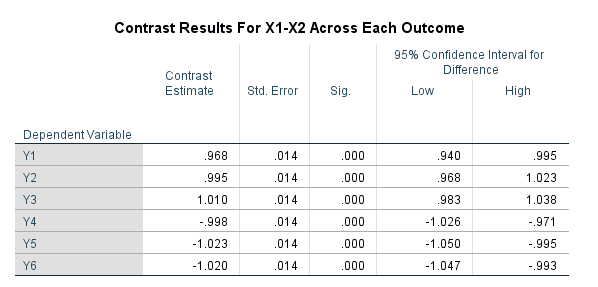Difference in independent effects for multivariate analysis (SPSS)

For some reason my various posts on testing differences in coefficients are fairly high in google search results. Arden Roeder writes in with another related question on this:

Good evening Dr. Wheeler,

I am a PhD candidate at the University of Oklahoma working on the final phases of data analysis for my dissertation. I found an article on your website that almost answers a question I have about a potential statistical test, and I’m hoping you might be able to help me fill in the gaps.

If you are willing to help me out, I would greatly appreciate it, and if not, I completely understand!

Here’s the setup: I have two independent variables (one measured on a 6-point Likert scale, the other on a 7-point) and six dependent variables. I have hypothesized that IV1 would be a stronger predictor of three of the DVs, and that IV2 would be a stronger predictor of the other three DVs. I ran multiple linear regression tests for each of the DVs, so I have the outputs for those. I know I can compare the standardized betas just to see strength, but what I’d like to know is how I can determine whether the difference between the beta weights is significant, and then to assess this for each of the six DVs.

From reading through your post, it seems like the fourth scenario you set up is quite close to what I’m trying to do, but I’m not sure how to translate the covariance output I have (I’m using SPSS) to what you’ve displayed here. Can I simply square the standard errors I have to get the numbers on the diagonal, and then grab the covariance from the SPSS output and solve accordingly? (I also reviewed your writing here about using the GLM procedure as well, but can’t seem to align my outputs with your examples there either.)

Here’s a sample of the numbers I’m working with:Any insights you can offer on 1) whether this is the right test to give me the answers I’m looking for about whether the betas are significantly different and 2) how to set up and interpret the results correctly would be a tremendous help.

For 1, yes I think this is an appropriate way to set up the problem. For 2, if sticking to SPSS it is fairly simple syntax in GLM:

*****************************.
*Contrasts with X1 - X2 effect across the variables.
GLM Y1 Y2 Y3 Y4 Y5 Y6 WITH X1 X2
/DESIGN=X1 X2
/PRINT PARAMETER
/LMATRIX = "T1"
X1  1
X2 -1.
*****************************.

To get this to do the standardized coefficients, Z-score your variables before the GLM command (this is assuming you are estimating a linear model, and not a non-linear model like logit/Poisson). (I have full simulation SPSS code at the end of the post illustrating.)

Note that when I say the covariance between beta coefficients, this is NOT the same thing as the covariance between the original metrics. So the correlation matrix for X1 vs X2 here does not give us the information we need.

For 2, the reporting part, you can see the Contrast results K matrix table in SPSS. I would just transpose that table, make a footnote/title this is testing X1 – X2, and then just keep the columns you want. So here is the original SPSS contrast table for this result:And here is how I would clean up the table and report the tests:SPSS Simulation Code

Here I just simulate independent X’s, and give the Y’s consistent effects. You could also simulate multivariate data with specified correlations if you wanted to though.

****************************************************.
* Simulated data to illustrate coefficient diff.
* tests.
SET SEED 10.
INPUT PROGRAM.
LOOP #i = 1 TO 10000.
COMPUTE Id = #i.
END CASE.
END LOOP.
END FILE.
END INPUT PROGRAM.

COMPUTE X1 = RV.NORMAL(0,1).
COMPUTE X2 = RV.NORMAL(0,1).
COMPUTE Y1 = 1.5*X1 + 0.5*X2 + RV.NORMAL(0,1).
COMPUTE Y2 = 1.5*X1 + 0.5*X2 + RV.NORMAL(0,1).
COMPUTE Y3 = 1.5*X1 + 0.5*X2 + RV.NORMAL(0,1).
COMPUTE Y4 = 0.5*X1 + 1.5*X2 + RV.NORMAL(0,1).
COMPUTE Y5 = 0.5*X1 + 1.5*X2 + RV.NORMAL(0,1).
COMPUTE Y6 = 0.5*X1 + 1.5*X2 + RV.NORMAL(0,1).
EXECUTE.

*Contrasts with X1 - X2 effect across the variables.
GLM Y1 Y2 Y3 Y4 Y5 Y6 WITH X1 X2
/DESIGN=X1 X2
/PRINT PARAMETER
/LMATRIX = "Contrast X1 - X2"
X1  1
X2 -1.

*And here is an example stacking equations and using EMMEANS.
*Stacking equation approach, can work for various.
*Generalized linear models, etc.
VARSTOCASES
/MAKE Y FROM Y1 TO Y6
/Index Outcome.

GENLIN Y BY Outcome WITH X1 X2
/MODEL Outcome X1 X2 Outcome*X1 Outcome*X2
/CRITERIA COVB=ROBUST
/REPEATED SUBJECT=Id CORRTYPE=UNSTRUCTURED
/EMMEANS TABLES=Outcome CONTROL= X1(1) X2(-1).
****************************************************.